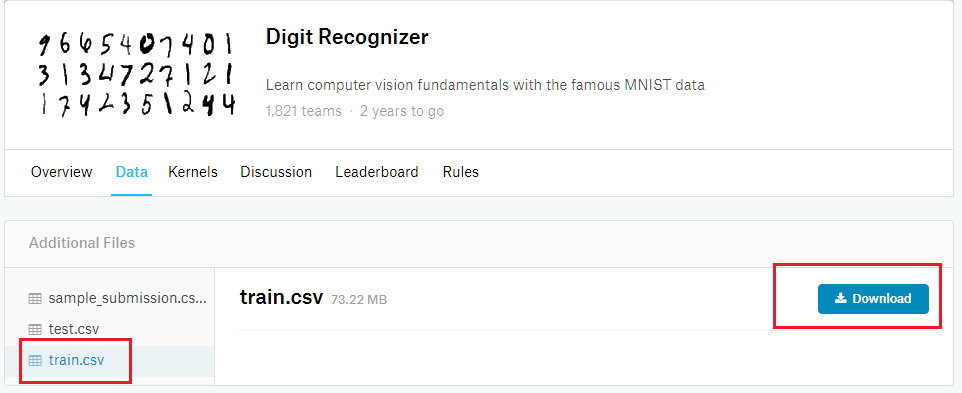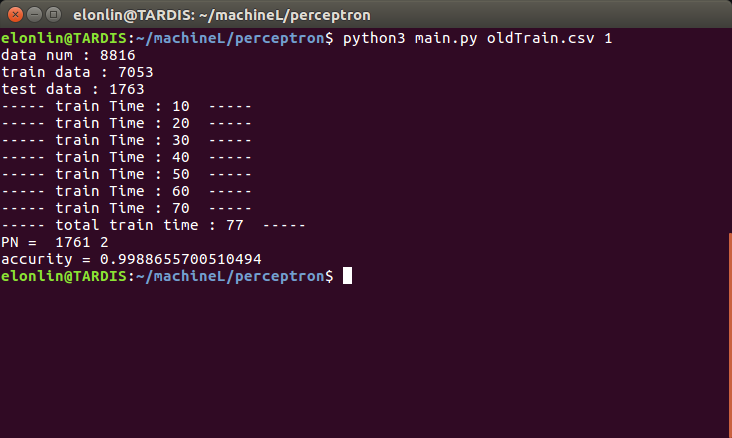@ArrowLLL 2017-08-14T14:20:53.000000Z 字数 6046 阅读 1485

# 感知机学习算法实践

Elon Lin

## 概述

• 系统 : ubuntu 16.04
• python版本： 3.5.1
• 为了理解原理自己造轮子，不使用拓展包

.
├── data.py
├── main.py
├── oldTrain.csv
├── perceptron.py
├── test.py
└── tree.txt

0 directories, 6 files

.
├── ans.csv
├── data.py
├── main.py
├── oldTrain.csv
├── perceptron.py
├── pycache
│     ├── data.cpython-35.pyc
│     ├── perceptron.cpython-35.pyc
│     └── test.cpython-35.pyc
├── test.csv
├── test.py
├── train.csv
└── tree.txt

1 directory, 12 files

## 数据来源与处理#!usr/bin/env python3# -*- coding:utf-8 -*-import sysimport randomdef get01(fileName) :    '''    fileName中含有的是带有标签0-9的数据，该函数用于将fileName中的2-9的数据    去除,只留下0和1的数据    '''    data = []    with open(fileName) as f:        lines = f.read().split()        tag = lines        for line in lines[1:] :            if line in ('1', '0') :                data.append(line)    return tag, datadef getData(args) :    '''    用于获取args文件中的0和1的数据，并将数据按照2:8分为数据集和测试集，以及测试集对应的    答案放入相应的文件 "train.csv", "test.csv", "ans.csv"    '''    # 获取只包含0和1的数据集    tags, lines = get01(args)    lineNum = len(lines)    # 随机取8:2的数据    testDataSet = set(random.sample(range(lineNum), (int)(lineNum / 5)))    # 创建相应的文件    train = open('train.csv', 'w+')    train.write(tags + '\n')    test = open('test.csv', 'w+')    tags.replace('label', 'case')    test.write(tags + '\n')    answer = open('ans.csv', 'w+')    answer.write("case,label\n")    # 写入文件    c = 0    for i, line in zip(range(lineNum), lines) :        label = '+1' if line == '1' else '-1'        if i in testDataSet :            answer.write("%s,%s\n" % (c, label))            test.write(str(c) + line[1:] + '\n')            c += 1        else :            line = label + line[1:]            train.write(line + '\n')    # 在终端输出分配结果    print ('data num : ' + str(lineNum))    print ('train data : ' + str(lineNum - len(testDataSet)))    print ('test data : ' + str(len(testDataSet)))    train.close()    test.close()    return 'train.csv', 'test.csv', 'ans.csv'if __name__ == '__main__' :    if len(sys.argv) == 2 :        oldFile = sys.argv    else :        oldFile = 'oldTrain.csv'    print (getData(oldFile))

data.py 的作用就是将原本的 oldTrain.csv 中的数据按照 8:2 的比例分为 train.csvtest.csv，以及 test.csv 对应的label的答案 ans.csv 。由于是随机取数据，故该代码可以重用。每运行一次可以由原来的数据生成不同的训练集和测试集。

## 感知机模型训练

#!usr/bin/env python3# -*- coding:utf-8 -*-w, b, eta = [], 0, 1def L(x) :    '''    函数 L(x) = w .* x + b    '''    return sum(wv * xv for (wv, xv) in zip(w, x)) + bdef findWrong(dataSet) :    '''    找到数据集中的误分类点，如果没有找到返回 None，找到则返回    一个元组 (误分类结果 y, 该误分类点的特征向量 x)    '''    t = 0    for (y,x) in dataSet :        t += 1        if L(x) * y <= 0 :            return (y, x)    return Nonedef sgd(data) :    '''    利用传入的误分类点使用梯度下降法极小化目标函数 :        w = w + (eta * y) .* x        b = b + eta * y    '''    global w, b, eta    y, x = data    w = [dw + eta * y * dx for (dw, dx) in zip(w, x)]    b = b + eta * ydef train(fileName, _eta = 1) :    '''    训练过程函数，感知机要求训练数据保证线性可分，采用的是原始形式的感知机学习算法    '''    # 取出对应csv按文件中的数据，并存入列表dataSet    # 列表中的单个数据以二元组形式存储，（标签， 特征向量）    f = open(fileName)    data = f.read().split()    column_names = data.split(',')    f.close()    dataSet = []    for singleData in data[1:] :        singleData = list(map(int, singleData.split(',')))        dataSet.append((singleData, singleData[1:]))    # 初始化全局变量 ：权值向量w, 偏置b, 学习率eta    global w, b, eta    eta = _eta    w = [0 for i in range(len(dataSet))]    trainTime = 0    # 训练过程，先找到一个误分类点，然后用sgd函数进行修正，    while True :        selectData = findWrong(dataSet)        if selectData == None : break        sgd(selectData)        trainTime += 1        if trainTime % 10 == 0 :            print ("----- train Time :", trainTime, " -----")    # 输出训练次数，即误分类次数    print ("----- total train time :", trainTime, " -----")    return w, bif __name__ == '__main__' :    w, b = train('train.csv')    print ("w :", w)    print ("b = ", b)

perceptron.py 即为训练模型的代码实现。采用的是感知机学习算法的原始形式，为了查看训练过程，每误分类10次就输出一次训练次数。

train() 函数中使用 findWrong() 函数找到误分类点，然后用 sgd() 函数利用梯度下降法极小化目标函数；findWrong() 函数中又调用 L(data) 函数得到目标函数值。

## 对测试集进行测试

#!usr/bin/env python3# -*- coding:utf-8 -*-w, b = [], 0def test(x) :    '''    按照模型 y = w .* x + b 计算对应数据的类别        y值非负返回为 +1        y值为负则返回 -1    '''    global w, b    L = sum(wv * xv for (wv, xv) in zip(w, x)) + b    return  1 if L >= 0 else -1def testFile(fileName, dw, db) :    '''    从测试文件fileName中读取测试集的特征，利用dw和db构成的感知机模型对    fileName中的测试数据进行分类    '''    global w, b    w, b = dw, db    # 读取数据    with open(fileName) as f :        testData = f.read().split()    # 使用 test()函数对数据进行分类，并将结果存入testResult列表    testResult, column_names = {}, testData    for data in testData[1:] :        data = data.split(',')        label = data        result = test(map(int, data[1:]))        testResult[label] = result    # 返回结果,为字典类型{label : result}    return testResultif __name__ == '__main__' :    dw = [0 for i in range(28 * 28)]    testResult = testFile('test.csv', dw, 0)    for label, result in testResult.items() :        if(result == -1) :            print (label, ":", result)

## 主函数main

main.py 文件将上述3份代码结合起来，将原来的 oldTrain.csv 进行剔除选择测试集和训练集，然后训练，然后测试，最终输出结果。

#!usr/bin/env python3# -*- coding:utf-8 -*-import dataimport perceptronimport testimport os, sysdef dealAns(ansFile) :    '''    读取csv文件ansFile，并进行处理获得结果字典{case : resultLabel}    '''    with open(ansFile) as f :        ansSet = f.read().split()        answer, column_names = {}, ansSet.split(',')        for ans in ansSet[1:] :            ans = ans.split(',')            answer[ans] = int(ans)    return answerdef main(dataFileName, eta) :    '''    传入参数包括原本包含0-9这10个数字的文件集合dataFileName, 以及预先传入的学习率eta值    在终端输出最终的测试结果，包括正确分类和错误分类的数量以及分类的正确率    '''    # 调用 data.getData 对数据集清洗并按照8 ：2分集    trainFile, testFile, ansFile = data.getData(dataFileName)    # 使用perceptron.train利用训练集训练感知机模型    w, b = perceptron.train(trainFile, eta)    # 调用 test.testFile 对测试文件中的数据进行测试，传入相应的w值和b值    testResult = test.testFile(testFile, w, b)    # 读取ans.csv文件返回一个字典 {case : label}    answer = dealAns(ansFile)    # 输出测试结果    positive, negative = 0, 0    for label, result in testResult.items() :        if answer[label] == result :            positive += 1        else :            negative += 1    print ("PN = ", positive, negative)    print ("accurity =", positive / (positive + negative))if __name__ == '__main__' :    argv = sys.argv    if(len(argv) == 3) :        main(argv, int(argv))    else :        'parame error !!'

## 运行过程与结果• 私有
• 公开
• 删除